#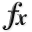N-D Test Functions H¶

class go_benchmark.Hansen(dimensions=2)

Hansen test objective function.

This class defines the Hansen global optimization problem. This is a multimodal minimization problem defined as follows:Here,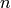represents the number of dimensions andfor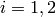.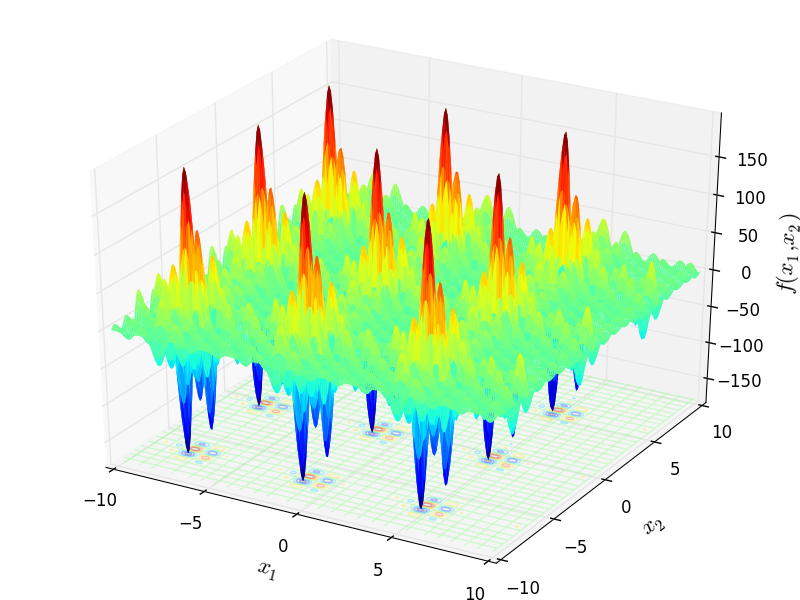Two-dimensional Hansen function

Global optimum:for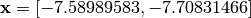.

class go_benchmark.Hartmann3(dimensions=3)

Hartmann3 test objective function.

This class defines the Hartmann3 global optimization problem. This is a multimodal minimization problem defined as follows: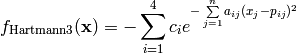Where, in this exercise: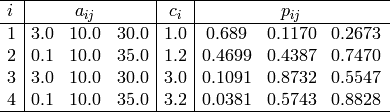Here,represents the number of dimensions and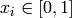for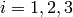.

Global optimum: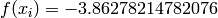forclass go_benchmark.Hartmann6(dimensions=6)

Hartmann6 test objective function.

This class defines the Hartmann6 global optimization problem. This is a multimodal minimization problem defined as follows:Where, in this exercise:Here,represents the number of dimensions andfor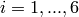.

Global optimum: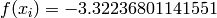for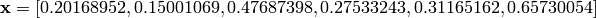class go_benchmark.HelicalValley(dimensions=3)

HelicalValley test objective function.

This class defines the HelicalValley global optimization problem. This is a multimodal minimization problem defined as follows: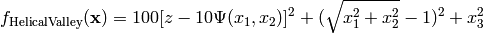Where, in this exercise: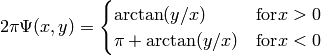Here,represents the number of dimensions and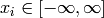for.

Global optimum: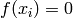for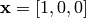class go_benchmark.HimmelBlau(dimensions=2)

HimmelBlau test objective function.

This class defines the HimmelBlau global optimization problem. This is a multimodal minimization problem defined as follows: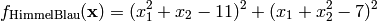Here,represents the number of dimensions and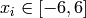for.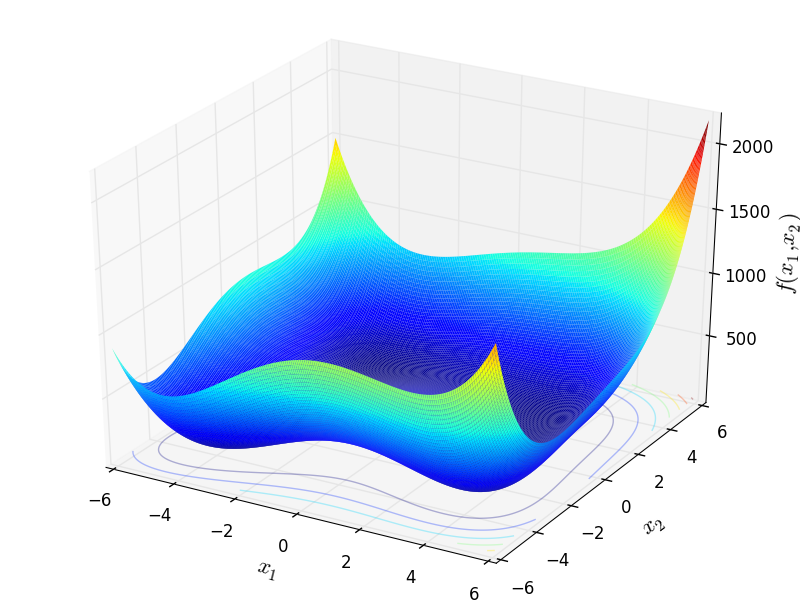Two-dimensional HimmelBlau function

Global optimum:forclass go_benchmark.HolderTable(dimensions=2)

HolderTable test objective function.

This class defines the HolderTable global optimization problem. This is a multimodal minimization problem defined as follows: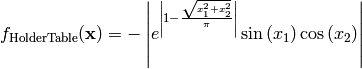Here,represents the number of dimensions andfor.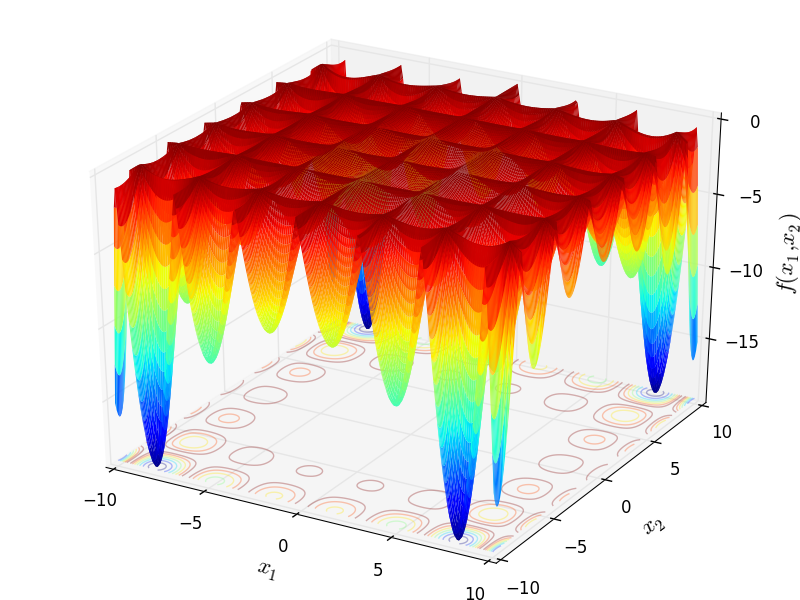Two-dimensional HolderTable function

Global optimum:for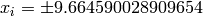forclass go_benchmark.Holzman(dimensions=3)

Holzman test objective function.

This class defines the Holzman global optimization problem. This is a multimodal minimization problem defined as follows:Where, in this exercise:Here,represents the number of dimensions and.

Global optimum:for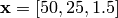class go_benchmark.Hosaki(dimensions=2)

Hosaki test objective function.

This class defines the Hosaki global optimization problem. This is a multimodal minimization problem defined as follows: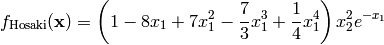Here,represents the number of dimensions and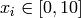for.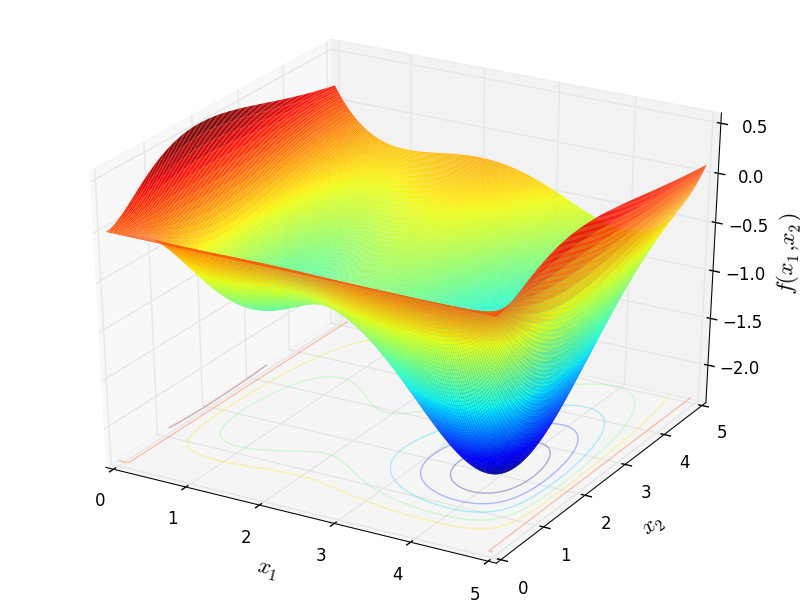Two-dimensional Hosaki function

Global optimum:for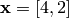.

#### Previous topic

N-D Test Functions G

#### Next topic

N-D Test Functions I